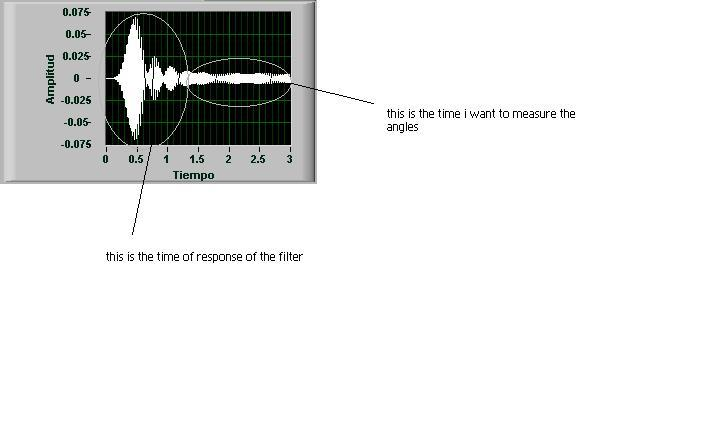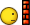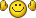# phase angle between two signals... OMG!

## Recommended Posts

Ok, first of all i want to say that is my pleassure to have this trouble and to have to fix it my self, so i don't want to sound like those students that actually want their homeworks done... it is just that perhaps i need another point of view ...So here is the deal, i have a 3-phasic electric system, of currents, the currents came out from a filter so, ignoring the response of the filter, i have to meassure the phase angles between them...

i know there are downloadable modules to do this, but the fact is that the time they use to meassure things like RMS values or else... it is a trouble cuz i have this response in my signalwell... let's pretend that i save my signals, the 3 of them, in .xls, and that i am going to errase somehow the non-stable part of them, just to keep the stable part of my signals, eventhough the "sub vi's" like "EPM_Phasor.vi" or "EPM_Phasor diagram"... i kind of bielieve that they are telling me lies... because they are triyin to meassure angles of current or voltage of the same phase, so those are never going to be more than 90°, but , in my sistem i may have 120° of separation between phases...

so i did this two things:

the first i tried... to use some trigonometrics with the RMS value of my signals, and then make a subbstraction of the signals, and take the RMS value of that too... in order to have a triangle, wich sides i know... whit Cosin Law, i'm able to see the angle, how do i know if the angle is positive or negative???, how do i know if i'm getting the supplementary or complementary angle or if i'm gettin the actual????

i finaly have the angles.... but i don't know if they are positive or negative...

my second try.... i used the RMS value and the DC value to calcule as if it was Power Factor the angle between the two currents... i have the same trouble because power factor is "Cos" if that angle...

but i'm mexican, and even if i try i do not, i repeat, i do not speak english... so greetings..angulo para armonicas.vi

##### Share on other sites

Ok, first of all i want to say that is my pleassure to have this trouble and to have to fix it my self, so i don't want to sound like those students that actually want their homeworks done... it is just that perhaps i need another point of view ...So here is the deal, i have a 3-phasic electric system, of currents, the currents came out from a filter so, ignoring the response of the filter, i have to meassure the phase angles between them...

i know there are downloadable modules to do this, but the fact is that the time they use to meassure things like RMS values or else... it is a trouble cuz i have this response in my signalbut i'm mexican, and even if i try i do not, i repeat, i do not speak english... so greetings..If you know the frequency of your signals then you could emulate a two-phase lockin in software. Take a section of your signal with say > 20 periods in it. and make two copies of it. Then for each of your three phases, multiply one sample by sine and average it and then multiply the other sample by cosine and average it. Take the two averages for each phase, feed them through a two input inverse tangent and get the phase ang;e for each phase (referred to an arbitary angle, but you are only interested in the difference between these angles). Although your singal may not be stable over the period you sample it over, so long as you use the same sample for both sine and cosine multiplications it should be ok.

If you don't know the frequency then you can probably extract the information from a fourier transform of the signal, finding the biggest peak in the appropriate frequency range for your expected signals and then useing the ratio of the eal and imaginary parts of the FT to work out phase angles.

This all assumes that I've (a) understood the question and (b) not had a brain storm.

##### Share on other sites

Just a rough scetch of a different idea. Using an anlog oszilloscope, you would use the 1st signal for the X and the 2nd signal for the Y channel. If both are sine signals of the same frequency with a phase difference, you'd get an ellipsis. Then get the distance between the crossings on the X-axis and the Y-axis respectively. I don't remember the exact formula, but I think the phase was simply sine of the ratio of both, easy math I did at age 17.

If you apply this to the signals you have, just get all crossings of the signals at 0A and take the value of the other signal.

Using lockin-technology (mentioned above) is also nice. You need to shift 1 of the signals by 90° (so you actually need to measure the frequency first). Then multiply the 2nd signal by the unshifted as well as the shifted signal. Sum both signals. Then do the arctan (?) on the ratio to obtain the phase.

I'd also search for phase-locking, phase-(de)modulation and phase-measurement on wikipedia. There might be some better techniques than my first ideas ('cause in both situations I was more/also interested in the amplitude).

Felix

##### Share on other sites

Just a rough scetch of a different idea. Using an anlog oszilloscope, you would use the 1st signal for the X and the 2nd signal for the Y channel. If both are sine signals of the same frequency with a phase difference, you'd get an ellipsis. Then get the distance between the crossings on the X-axis and the Y-axis respectively. I don't remember the exact formula, but I think the phase was simply sine of the ratio of both, easy math I did at age 17.

If you apply this to the signals you have, just get all crossings of the signals at 0A and take the value of the other signal.

Does this still work if the amplitude is also varying with time ? I guess if you do lots of measurements and average over time it's ok...

##### Share on other sites

Does this still work if the amplitude is also varying with time ? I guess if you do lots of measurements and average over time it's ok...

No, I'd say you need to calculate the phase for each full circle. Then average the results.

But there are certainly exceptions to this. If you had a constant sine (you actually did exclude this) with a lot of noise (or very bad SNR, averaging dX and dY first and then calculating the phase would be better. I have not a mathematical model for this, but I'd say a very important guidline is to look at the dividers in any formula. If they are close (in the distance of the noise, e.g. 3sigma) to zero, the noise can produce values close to 0 -> the whole term becomes inifinite and dominates any averaging.

To my experience, doing signal analysis is a fascinating experimental science. In case you don't have a real measurement ('cause the setup is unfinished), use some simulated signals. At various processing steps, just plot time signal and a FFT. It will always be a good learning experience.

Sea-story: My latest setup I showed to a PhD'ed physicist and got the response: 'wow, like in the textbook'. And the student writing his master-thesis got very-very big eyes when we didn't see any signal anymore in the FFT and I recovered it using lockin technique.Felix

##### Share on other sitesNo WAY!!!!

I always thought that if I make an average of a Sine Wave.. I would get Zero… or something as close as zero as possible….

Gb119, I apologize because I actually thought that you were Crazy at first but it actually works… and works perfectly…

This method is awesome… I wonder what’s the math behind this… are you guys warlocks or something??? Is it witchery???

I keep searching about it with the name “phase-locking” and the other names Black pearl gave me, and I found that is usually done in Labview..

they use the Hilbert Transformation to make the sine wave a Cosine wave.... well kind of... and then use the statisitic average and to the inverse of the Tangent they use the complex Numbers convertions....

So this is what finally was done

i just don't know what is the "x 2" for... and at first i use the "Normalize Waveform" to see if the filter response is something not to care about...

well i'm between afraid and impressed, i actually learn something new tanks a lot guys...

Edited by 335_x
##### Share on other sitesNo WAY!!!!

I always thought that if I make an average of a Sine Wave.. I would get Zero… or something as close as zero as possible….

Gb119, I apologize because I actually thought that you were Crazy at first but it actually works… and works perfectly…

This method is awesome… I wonder what’s the math behind this… are you guys warlocks or something??? Is it witchery???

No, just an experimental physicist.Hmm, on second thoughts, in some places and times, the two would be more or less the same thing !

The maths behind phase sensitive detection is quite simple really - I teach it to my undergraduates when I explain how a lockin amplifier works. Basically if you take the Fourier transform of your input signals then you end up with a bunch of sine waves at different frequencies, each with their own amplitude and phase:

S=a_0+a_1 sine (w_1 t + f_1) + a_2 sine (w_2 +f_2)+...a_n sine (w_n t +f_n)

When you multiply by a sine wave of known frequency w_r you can use the rig idenity:

sin A sin B = 1/2(cos(A-B)-cos(A+B)), for each term in S where w_n!= w_r you are going to have terms that involve cos (something t). Averaging those over time and indeed you get zero. However, the one term for which w_n=w_r, you are going to get:

1/2(cos({w_r-w_r }t-f_r)-cos(2w_r t + f_r)) which averaged over time gives 1/2 cos f_r.

Do the same with multiplying by cos w_r t and you get 1/2 sin f_r time averaged and you're away.

I suspect without thinking too hard that your x2 is just correcting for the factor 1/2 that comes from the trig identity.

##### Share on other sites

This method is awesome… I wonder what’s the math behind this… are you guys warlocks or something??? Is it witchery???

Well, this is the fun (I was talking about that before) you have when doing signal analysis.

The Hilbert Transformation is the Phase Shifter of the Lockin (sin -> -cos = 90°). The multiplication is the demodulation (some more on this next). The mean is just a low-pass filter.

Now you can look at the FFT at the different steps: The demodulation is frequency shifting your signal to |f1-f2| and f1+f2 (where f1,f2 are the frequencies of both signals). As both frequencies are equal f1-f2 is actually giving you a DC signal.

Now just low-pass filter the reult to obtain the DC signal.Felix

## Join the conversation

You can post now and register later. If you have an account, sign in now to post with your account.×   Pasted as rich text.   Paste as plain text instead

Only 75 emoji are allowed.

×   Your previous content has been restored.   Clear editor

×   You cannot paste images directly. Upload or insert images from URL.

×
×